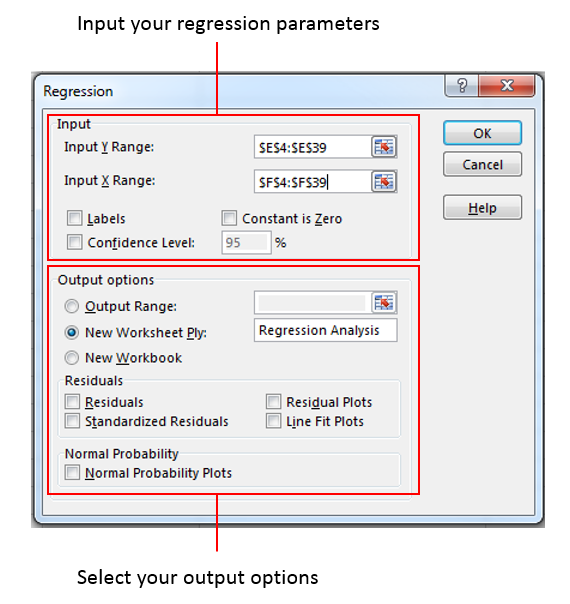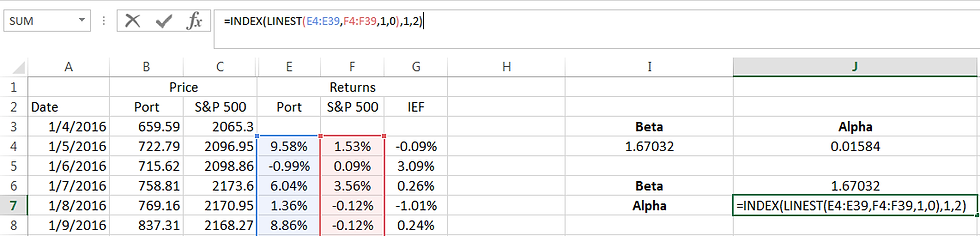top of pageSearch
• Eng Guan

# Linear Regression – Finding Alpha And Beta

Linear regression is a widely used data analysis method. For instance, within the investment community, we use it to find the Alpha and Beta of a portfolio or stock. If you are new to this, it may sound complex. But it is, in fact, simple and fairly easy to implement in Excel. And this is what this post is about.

## Linear Regression

Linear regression is, as the name suggests, about investigating linear relations between an outcome and one or more inputs. In mathematical terms, we call this outcome the dependent variable and the inputs the independent variables. As we vary the inputs, we want to observe their impact on the outcome.

The central idea is to determine the best-fitted straight line, plane, or hyperplane through the data points depending on how many input variables (dimensions) there are. In math, we express them as:

Y = m1 X1 + m2 X2 + …. mn Xn + c

Where:

1. Y is the dependent variable.

2. m1, … , mn are the exposure (gradients) to the respective independent variables Xs.

3. X1, …, Xn are the independent variables.

4. c is the constant (intercept).

Given the Ys and Xs, linear regression tells you what are the ms (gradients) and c (constant).

I am not going into the academic details. If you took high school math, this should not be something unfamiliar. In case you want a more rigorous treatment of the topic, you can look up Wikipedia. Else, let’s see how we can use Excel to find the Alpha and Beta of a portfolio.

## Finding the Alpha and Beta of a Portfolio

The equation below is what we want to fit.Rp is the portfolio return, Rm is the market return and Rf is the risk-free rate.

Let’s say we have the monthly returns of a US portfolio and we want to know its Alpha and Beta against the S&P 500 index. To simplify matters, let's set the risk-free rate, Rf, to zero.

##### Method 1: Using Slope() and Intercept()

For 2-dimensional fitting problems like finding the Alpha and Beta of a portfolio, you can use the SLOPE() and INTERCEPT() functions in Excel.

1. Use SLOPE() to find the Beta

SLOPE(Y-series, X-series)

This function calculates the gradient of the best-fitted line when we plot Y against X. In this case, Y is the monthly portfolio returns and X is the monthly S&P 500 returns.2. Use INTERCEPT() to find the Alpha

INTERCEPT(Y-series, X-series)

Now, switch SLOPE() with INTERCEPT(). The inputs remain the same. And there you go, you get the Alpha of the portfolio.##### Method 2: Using Data Analysis

There is a data tab at the top menu in Excel. If you click on it, you should be able to see the Data Analysis tool if you have the Analysis ToolPak enabled.Click on Data Analysis. This brings up a small window of options.Select Regression and click OK. This brings up a window for you to fill up the regression parameters and options.For inputs, we put the monthly portfolio returns as Y and monthly S&P 500 returns as X. As we do not have a label on the first row of our data, we leave “Labels” unchecked. We also leave “Constant is Zero” unchecked since are not looking to force the intercept of the fitted line to zero. As for “Confidence Level”, it tells Excel to display the lower and upper bounds of the estimated parameters that capture the specified percentage of all the estimates. By default, Excel will always show you the bounds for a 95% confidence interval.

In the outputs section, we can ask Excel to export the results into a specified range, a new worksheet, or a new workbook. It is really up to you. For this case, I opt to print the analysis results to a new worksheet named Regression Analysis. And moving on, we have options to output data on the residuals, i.e. the differences between Y as predicted on the fitted line and the actual Y. And finally, there is an option to print a normality plot which gives us a sense of how well the actual Y data fits a normal distribution. The aim here, however, is not to do an analysis. So we will not select any of these.

Now, click OK.Excel prints the results to a page called Regression Analysis as per what we instruct. For ease of reference, I highlighted the cells we want in yellow. The Alpha is the Intercept Coefficient and the Beta is the X Variable 1 Coefficient.

For those who want to know more about how to interpret the various outputs, you can visit here.

##### Method 3: LINEST() function

This is the most versatile method. LINEST() can perform regression against multiple independent variables. As it is also a built-in function, you can drag it through a time series to do a rolling regression, unlike the data analysis tool.

LINEST(Y-series, X-series, Constant, Stats)

LINEST() takes in the same inputs as SLOPE() and INTERCEPT(). In addition to that, we can specify if we want to calculate the constant (intercept) and whether we want to output additional statistics. That seems like an awful amount of data to display. To handle that, LINEST() returns an array in the following form:m1, … , mn-1 are the exposure (gradients) to the respective independent variables Xs. Note that it is displayed in reverse order. b is the constant or your intercept while the rest are additional statistics on the estimated parameters which I will not be covering.

In our case, there are only 2 elements of interest – Beta (Slope) and Alpha (Intercept). To make LINEST display both elements, we select 2 consecutive cells I4 to J4, press F2 to enter the formula, and hit Ctrl+Shift+Enter.If we want the numbers displayed in other ways like in a column, we can make use of the INDEX() function. Since LINEST() output an array, we can nest LINEST() within INDEX() to extract the element we want. Beta is in row 1 column 1 (1,1) and Alpha is in row 1 column 2 (1,2). See how it is done below.## Conclusion

These are a few ways you can find the Alpha and Beta of a stock or portfolio given their return series. SLOPE() and INTERCEPT() are the simplest way. They are sufficient for cases with a single independent variable such as this and if you do not require further analysis. Then we have the data analysis tool that provides a more in-depth statistical analysis of the parameter estimates and is able to perform regression with multiple independent variables. This method is, however, not suitable for generating rolling regression estimates. Finally, there is the LINEST() function. It has the flexibility of an Excel function, works on multiple independent variables, and is capable of providing statistical information on the estimates.

Want to know more about AllQuant?